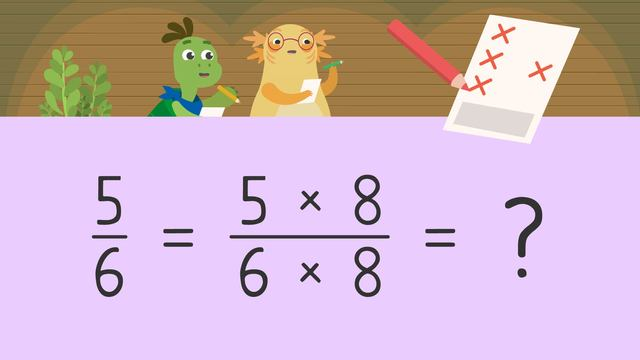# Generate Equivalent FractionsRating

Ø 3.3 / 6 ratings
The authorsTeam Digital
Generate Equivalent Fractions
CCSS.MATH.CONTENT.4.MD.A.1

## Equivalent Fractions – Definition

In this learning text we will be looking at equivalent fractions and how to find equivalent fractions. You may already know from our previous videos what a fraction is. Let’s look at the recap of the definition of what fractions and equivalent fractions are.

• Fractions always represent a part of a whole. For example, if a pizza is divided into four equal pieces, one piece is called $\frac{1}{4}$ of a whole pizza.

• Equivalent fractions are fractions which have the same value but are represented with a different numerator and denominator.

Equivalent fractions are created by breaking a whole into smaller equal parts. For example, the equivalent fractions visual diagram below is showing a whole as a fraction of $\frac{2}{2}$ and another fraction which is still one whole as a fraction $\frac{8}{8}$. So, the fractions $\frac{2}{2}$ and $\frac{8}{8}$ are equivalent fractions.To create equivalent fractions, we can increase the number of pieces by multiplying the numerator and the denominator by the same n factor.

In mathematics, we use a multiplication expression to represent an equivalent fraction. The left-hand side of the expression represents our fraction and the right-hand side of the expression represents an equivalent fraction multiplied by n, where n represents any number. The equal sign shows that both sides of the expression are equal to each other. The bigger the number we multiply the fraction by, the more equal parts the whole has.original fraction how to find the equivalent fraction
$\frac{a}{b}$ $\frac{a x n}{b x n}$

Now, we can look at some examples for better understanding of equivalent fractions and after that you can practice equivalent fractions worksheet 4th grade or play equivalent fractions bingo.

## Equivalent Fractions – Example 1

Let’s look at our first fraction, which is $\frac{1}{2}$. If we multiply the numerator and the denominator by two, we have generated an equivalent fraction which is $\frac{2}{4}$. The size of these parts is different, but the size of the whole remains the same.fraction equivalent fraction
$\frac{1}{2}$ $\frac{2}{4}$

## Equivalent Fractions – Example 2

In our second example, we are looking at $\frac{3}{4}$. Let’s multiply the numerator and the denominator of the fraction by three, so we will get $\frac{9}{12}$. In this example, the n equals three.We can also use a different factor; this time let’s multiply the numerator and the denominator of $\frac{3}{4}$by six, so we will get an equivalent fraction which is $\frac{18}{24}$. This time, the n factor equals six.We can changethen factor for any other number, we just must remember to multiply the numerator and the denominator by the same n factor.

fraction equivalent fraction
$\frac{1}{2}$ $\frac{2}{4}$

## Equivalent Fractions – Summary

How do we generate or create an equivalent fraction? Look at the summary below:

• Equivalent fractions are fractions that have the same value but have different numbers in the numerators and denominators.
• Equivalent fractions are created by breaking a whole into smaller equal parts and having a greater number of pieces.
• We can increase the number of parts in a fraction by multiplying the numerator and the denominator by the same factor.
• Use the multiplication expression.
• Pick a number and then multiplying the numerator and denominator by the chosen factor.